JEE  >  Test: Comprehension Based Questions: Conic Sections

# Test: Comprehension Based Questions: Conic Sections

Test Description

## 14 Questions MCQ Test Maths 35 Years JEE Main & Advanced Past year Papers | Test: Comprehension Based Questions: Conic Sections

Test: Comprehension Based Questions: Conic Sections for JEE 2022 is part of Maths 35 Years JEE Main & Advanced Past year Papers preparation. The Test: Comprehension Based Questions: Conic Sections questions and answers have been prepared according to the JEE exam syllabus.The Test: Comprehension Based Questions: Conic Sections MCQs are made for JEE 2022 Exam. Find important definitions, questions, notes, meanings, examples, exercises, MCQs and online tests for Test: Comprehension Based Questions: Conic Sections below.
Solutions of Test: Comprehension Based Questions: Conic Sections questions in English are available as part of our Maths 35 Years JEE Main & Advanced Past year Papers for JEE & Test: Comprehension Based Questions: Conic Sections solutions in Hindi for Maths 35 Years JEE Main & Advanced Past year Papers course. Download more important topics, notes, lectures and mock test series for JEE Exam by signing up for free. Attempt Test: Comprehension Based Questions: Conic Sections | 14 questions in 30 minutes | Mock test for JEE preparation | Free important questions MCQ to study Maths 35 Years JEE Main & Advanced Past year Papers for JEE Exam | Download free PDF with solutions
 1 Crore+ students have signed up on EduRev. Have you?
Test: Comprehension Based Questions: Conic Sections - Question 1

### PASSAGE 1 Consider the circle x2 + y2 = 9 and the parabola y2 = 8x. They intersect at P and Q in the first and the fourth quadrants, respectively. Tangents to the curcle at P and Q intersect the x-axis at R and tangents to the parabola at P and Q intersect the x-axis at S. (2007 -4 marks) Q. The ratio of the areas of the triangles PQS and PQR is

Detailed Solution for Test: Comprehension Based Questions: Conic Sections - Question 1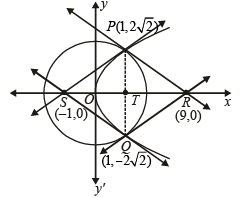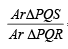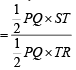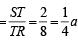Test: Comprehension Based Questions: Conic Sections - Question 2

### PASSAGE 1 Consider the circle x2 + y2 = 9 and the parabola y2 = 8x. They intersect at P and Q in the first and the fourth quadrants, respectively. Tangents to the curcle at P and Q intersect the x-axis at R and tangents to the parabola at P and Q intersect the x-axis at S. (2007 -4 marks) Q. The radius of the circumcircle of the triangle PRS is (2007 -4 marks)

Detailed Solution for Test: Comprehension Based Questions: Conic Sections - Question 2For ΔPRS,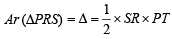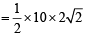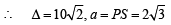b = PR = 6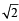, c = SR = 10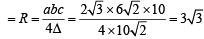Test: Comprehension Based Questions: Conic Sections - Question 3

### PASSAGE 1 Consider the circle x2 + y2 = 9 and the parabola y2 = 8x. They intersect at P and Q in the first and the fourth quadrants, respectively. Tangents to the curcle at P and Q intersect the x-axis at R and tangents to the parabola at P and Q intersect the x-axis at S. (2007 -4 marks) Q. The radius of the incircle of the triangle PQR is (2007 -4 marks)

Detailed Solution for Test: Comprehension Based Questions: Conic Sections - Question 3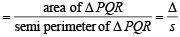We have a = PR  =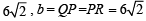c = PQ =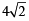and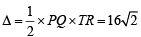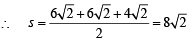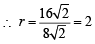Test: Comprehension Based Questions: Conic Sections - Question 4

PASSAGE 2

The circle x2 + y2 – 8x = 0 and hyperbola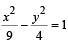intersect atthe points A and B. (2010)

Q. Equation of a common tangent with positive slope to the circle as well as to the hyperbola is

Detailed Solution for Test: Comprehension Based Questions: Conic Sections - Question 4

Any tangent to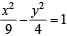is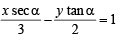It touches circle with center (4,0) and radius = 4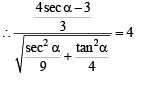⇒ 16 sec2α – 24 secα + 9 =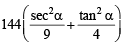⇒ 12 sec2α + 8 sec– 15= 0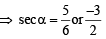but sec α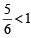is not possible

∴ sec α = –3/2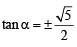∴  slope of tangent =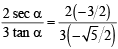.

(for +ve value of m)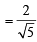∴ Equation of tangent is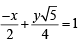or 2 x –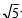y + 4=0

Test: Comprehension Based Questions: Conic Sections - Question 5

PASSAGE 2

The circle x2 + y2 – 8x = 0 and hyperbolaintersect atthe points A and B. (2010)

Q. Equation of the circle with AB as its diameter is

Detailed Solution for Test: Comprehension Based Questions: Conic Sections - Question 5

The intersection points of given circle x2 + y2 – 8x= 0...(1) .
and hyperbola 4 x2 –9y2 –36 = 0 ...(2)
can be obtained by solving these equations
Substituting value of y2 from eqn (1) in eqn (2), we get 4 x2 – 9(8x –x2) = 36 ⇒ 13x2 – 72x – 36= 0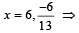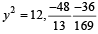(not possible)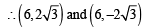are points of intersection.
So eqn of required circle is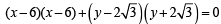⇒ x2 + 36 – 12 x +y– 12= 0

⇒ x2+ y2 – 12x + 24=0

Test: Comprehension Based Questions: Conic Sections - Question 6

PASSAGE 3
Tangents are drawn from the point P(3, 4) to the ellipse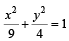touching the ellipse at points A and B. (2010)

Q. The coordinates of A and B are

Detailed Solution for Test: Comprehension Based Questions: Conic Sections - Question 6

Tangent to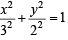at  the point (3 cosθ, 2 sinθ) is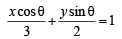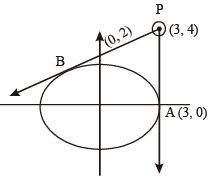As it passes throught (3,4), we get cosθ + 2 sinθ=1
⇒ 4 sin2θ = 1 + cos2θ – 2 cosθ
⇒ 5cos2θ – 2cosθ – 3= 0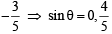∴ Required points are A (3, 0) and B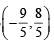Test: Comprehension Based Questions: Conic Sections - Question 7

PASSAGE 3
Tangents are drawn from the point P(3, 4) to the ellipsetouching the ellipse at points A and B. (2010)

Q. The orthocenter of the triangle PAB is

Detailed Solution for Test: Comprehension Based Questions: Conic Sections - Question 7

Let H be the  orthocentre of ΔPAB, then as BH ⊥ AP, BH is a horizontal line  through B.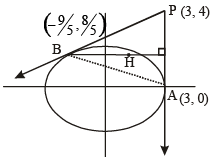∴ y- cordinate of B = 8/5

Let H has coordinater (α, 8 5)

Then slope of PH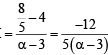and slope of AB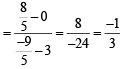But PH ⊥ AB ⇒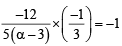⇒ 4 = –5α + 15 or α = 11/5

Hence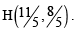Test: Comprehension Based Questions: Conic Sections - Question 8

PASSAGE 3
Tangents are drawn from the point P(3, 4) to the ellipsetouching the ellipse at points A and B. (2010)

Q. The equation of the locus of the point whose distances from the point P and the line AB are equal, is

Detailed Solution for Test: Comprehension Based Questions: Conic Sections - Question 8

Clearly the moving point traces a parabola with focus at P(3, 4) and directrix as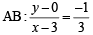or x + 3y – 3=0

∴ Equation of parabola is

(x– 3)2 + (y–4)2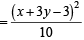or 9x2 + y2 – 6xy – 54x – 62y + 241= 0

Test: Comprehension Based Questions: Conic Sections - Question 9

PASSAGE 4

Let PQ be a focal chord of the parabola y2 = 4ax. The tangents to the parabola at P and Q meet at a point lying on the line y = 2x + a, a > 0.

Q. Length of chord PQ is (JEE Adv. 2013)

Detailed Solution for Test: Comprehension Based Questions: Conic Sections - Question 9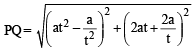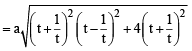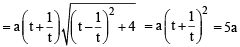Test: Comprehension Based Questions: Conic Sections - Question 10

PASSAGE 4

Let PQ be a focal chord of the parabola y2 = 4ax. The tangents to the parabola at P and Q meet at a point lying on the line y = 2x + a, a > 0.

Q. If chord PQ subtends an angle θ at the vertex of y2 = 4ax, then tan q =            (JEE Adv. 2013)

Detailed Solution for Test: Comprehension Based Questions: Conic Sections - Question 10

As PQ is the focal chord of  y2 = 4ax

∴ Coordinates of P and Q can be taken as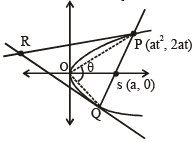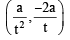P(at2 , 2at) and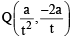Tangents at P and Q are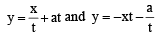which intersect each other  at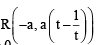As R lies on the y = 2x + a,  a > 0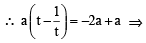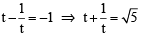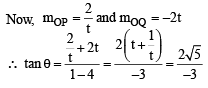Test: Comprehension Based Questions: Conic Sections - Question 11

PASSAGE 5
Let a, r, s, t be nonzero real numbers. Let P (at2, 2at), Q, R (ar2, 2ar) and S (as2, 2as) be distinct points on the parabola y2 = 4ax. Suppose that PQ is the focal chord and lines QR and PK are parallel, where K is the point (2a, 0) (JEE Adv. 2014)

Q. The value of r is

Detailed Solution for Test: Comprehension Based Questions: Conic Sections - Question 11

∵ PQ is a focal chord,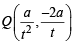Also QR || PK ⇒ mQR = mPK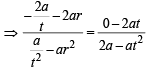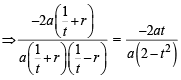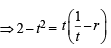[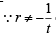otherwise Q will coincide with R]

⇒ 2 - t2 = 1- tr ⇒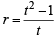Test: Comprehension Based Questions: Conic Sections - Question 12

PASSAGE 5
Let a, r, s, t be nonzero real numbers. Let P (at2, 2at), Q, R (ar2, 2ar) and S (as2, 2as) be distinct points on the parabola y2 = 4ax. Suppose that PQ is the focal chord and lines QR and PK are parallel, where K is the point (2a, 0) (JEE Adv. 2014)

Q. If st = 1, then the tangent at P and the normal at S to the parabola meet at a point whose ordinate is

Detailed Solution for Test: Comprehension Based Questions: Conic Sections - Question 12

Tangent at P is
ty = x+ at2 ....(i)
Normal at S sx + y = 2as + as3 ....(ii)

But given st = 1 ⇒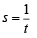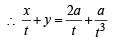⇒ xt2 = yt3 = 2at+ a

Putting value of x from equation (i) in above equation we get

⇒ t2 ( ty - at2) + yt3 = 2at+ a

⇒ (t3 + t3) y - at4 = 2at+ a

⇒ 2t3y = a (t4 + 2t2+1)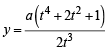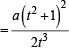Test: Comprehension Based Questions: Conic Sections - Question 13

PASSAGE 6
Let F1(x1, 0) and F2(x2, 0) for x1 < 0 and x2 > 0, be the foci of the ellipse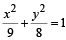Suppose a parabola having vertex at the origin and focus at F2 intersects the ellipse at point M in the first quadrant and at point N in the fourth quadrant.

Q. The orthocentre of th e triangle F1MN is (JEE Adv. 2016)

Detailed Solution for Test: Comprehension Based Questions: Conic Sections - Question 13

For ellipse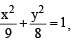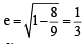∴ F1(– 1, 0) and F2 (1, 0) Parabola with vertex at (0, 0) and focus at F2(1, 0) is y2 = 4x.
Intersection points of ellipse and parabola are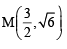and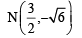For orthocentre of ΔF1MN, clearly one altitude is x-axis i.e. y = 0 and altitude from M to F1N is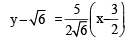Putting y = 0 in above equation, we get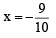∴ Orthocentre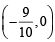Test: Comprehension Based Questions: Conic Sections - Question 14

PASSAGE 6
Let F1(x1, 0) and F2(x2, 0) for x1 < 0 and x2 > 0, be the foci of the ellipseSuppose a parabola having vertex at the origin and focus at F2 intersects the ellipse at point M in the first quadrant and at point N in the fourth quadrant.

Q. If the tangen ts to the ellipse at M an d N meet at R and the normal to the parabola at M meets the x-axis at Q, then the ratio of area of the triangle MQR to area of the quadrilateral MF1NF2 is   (JEE Adv. 2016)

Detailed Solution for Test: Comprehension Based Questions: Conic Sections - Question 14

For ellipse∴ F1(– 1, 0) and F2 (1, 0) Parabola with vertex at (0, 0) and focus at F2(1, 0) is y2 = 4x.
Intersection points of ellipse and parabola areandTangents to ellipse at M and N are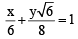and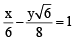Their intersection point is R (6, 0)

Also normal to parabola at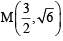is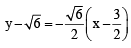Its intersection with x-axis is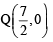Now ar (ΔMQR)  =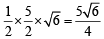Also area (MF1NF2) = 2 × Ar (F1MF2)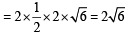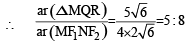## Maths 35 Years JEE Main & Advanced Past year Papers

111 docs|70 tests
 Use Code STAYHOME200 and get INR 200 additional OFF Use Coupon Code
Information about Test: Comprehension Based Questions: Conic Sections Page
In this test you can find the Exam questions for Test: Comprehension Based Questions: Conic Sections solved & explained in the simplest way possible. Besides giving Questions and answers for Test: Comprehension Based Questions: Conic Sections, EduRev gives you an ample number of Online tests for practice

## Maths 35 Years JEE Main & Advanced Past year Papers

111 docs|70 tests